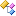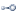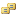﻿ Accord.Statistics.Analysis NamespaceAccord.Statistics.Analysis Namespace

Contains many statistical analysis, such as PCA, LDA, KPCA, KDA, PLS, ICA, Logistic Regression and Stepwise Logistic Regression Analyses. Also contains performance assessment analysis such as contingency tables and ROC curves.Classes
ClassDescriptionBaseDiscriminantAnalysis
Base class for Discriminant Analysis (LDA, QDA or KDA).CircularDescriptiveAnalysis
Descriptive statistics analysis for circular data.CircularDescriptiveMeasureCollection
Collection of descriptive measures.CircularDescriptiveMeasures
Circular descriptive measures for a variable.ConfusionMatrix
Binary confusion matrix for binary decision problems. For multi-class decision problems, please see GeneralConfusionMatrix.DescriptiveAnalysis
Descriptive statistics analysis.DescriptiveMeasureCollection
Collection of descriptive measures.DescriptiveMeasures
Descriptive measures for a variable.Discriminant

Represents a discriminant factor found during Discriminant Analysis, allowing it to be bound to controls like the DataGridView.

This class cannot be instantiated.DiscriminantAnalysisClass
Represents a class found during Discriminant Analysis, allowing it to be bound to controls like the DataGridView. This class cannot be instantiated.DiscriminantAnalysisClassCollection

Represents a collection of classes found in the Discriminant Analysis.

This class cannot be instantiated.DiscriminantCollection

Represents a collection of Discriminants factors found in the Discriminant Analysis.

This class cannot be instantiated.DistributionAnalysis
Distribution fitness analysis.GeneralConfusionMatrix
General confusion matrix for multi-class decision problems. For binary problems, please see ConfusionMatrix.GoodnessOfFit
Goodness-of-fit result for a given distribution.GoodnessOfFitCollection
Collection of goodness-of-fit measures.HazardCoefficient
Represents a Proportional Hazards Coefficient found in the Cox's Hazards model, allowing it to be bound to controls like the DataGridView. This class cannot be instantiated outside the LogisticRegressionAnalysis.HazardCoefficientCollection
Represents a collection of Hazard Coefficients found in the ProportionalHazardsAnalysis. This class cannot be instantiated.IndependentComponent
Represents an Independent Component found in the Independent Component Analysis, allowing it to be directly bound to controls like the DataGridView.IndependentComponentAnalysis
Independent Component Analysis (ICA).IndependentComponentCollection
Represents a Collection of Independent Components found in the Independent Component Analysis. This class cannot be instantiated.KernelDiscriminantAnalysis
Kernel (Fisher) Discriminant Analysis.KernelDiscriminantAnalysisPipeline
Standard regression and classification pipeline for LinearDiscriminantAnalysis.KernelPrincipalComponentAnalysis
Kernel Principal Component Analysis.LinearDiscriminantAnalysis
Linear Discriminant Analysis (LDA).LinearDiscriminantAnalysisPipeline
Standard regression and classification pipeline for LinearDiscriminantAnalysis.LinearRegressionCoefficient

Represents a Linear Regression coefficient found in the Multiple Linear Regression Analysis allowing it to be bound to controls like the DataGridView.

This class cannot be instantiated.LinearRegressionCoefficientCollection
Represents a Collection of Linear Regression Coefficients found in the MultipleLinearRegressionAnalysis. This class cannot be instantiated.LogisticCoefficient
Represents a Logistic Regression Coefficient found in the Logistic Regression, allowing it to be bound to controls like the DataGridView. This class cannot be instantiated outside the LogisticRegressionAnalysis.LogisticCoefficientCollection
Represents a collection of Logistic Coefficients found in the LogisticRegressionAnalysis. This class cannot be instantiated.LogisticRegressionAnalysis
Logistic Regression Analysis.MultinomialCoefficient

Represents a Multinomial Logistic Regression coefficient found in the multinomial logistic regression analysis allowing it to be bound to controls like the DataGridView.

This class cannot be instantiated.MultinomialCoefficientCollection
Represents a Collection of Multinomial Logistic Regression Coefficients found in the MultinomialLogisticRegressionAnalysis. This class cannot be instantiated.MultinomialLogisticRegressionAnalysis
Multinomial Logistic Regression AnalysisMultipleLinearRegressionAnalysis
Multiple Linear Regression AnalysisNestedLogisticCoefficient
Represents a Logistic Regression Coefficient found in the Logistic Regression, allowing it to be bound to controls like the DataGridView. This class cannot be instantiated outside the LogisticRegressionAnalysis.NestedLogisticCoefficientCollection
Represents a collection of Logistic Coefficients found in the LogisticRegressionAnalysis. This class cannot be instantiated.PartialLeastSquaresAnalysis
Partial Least Squares Regression/Analysis (a.k.a Projection To Latent Structures)PartialLeastSquaresFactor
Represents a Partial Least Squares Factor found in the Partial Least Squares Analysis, allowing it to be directly bound to controls like the DataGridView.PartialLeastSquaresFactorCollection
Represents a Collection of Partial Least Squares Factors found in the Partial Least Squares Analysis. This class cannot be instantiated.PartialLeastSquaresVariables
Represents source variables used in Partial Least Squares Analysis. Can represent either input variables (predictor variables) or output variables (independent variables or regressors).PrincipalComponent

Represents a Principal Component found in the Principal Component Analysis, allowing it to be bound to controls like the DataGridView.

This class cannot be instantiated.PrincipalComponentAnalysis
Principal component analysis (PCA) is a technique used to reduce multidimensional data sets to lower dimensions for analysis.PrincipalComponentCollection
Represents a Collection of Principal Components found in the PrincipalComponentAnalysis. This class cannot be instantiated.ProcrustedDataset
Class to represent an original dataset, its Procrustes form and all necessary data (i.e. rotation, center, scale...)ProcrustesAnalysis
Class to perform a Procrustes AnalysisProportionalHazardsAnalysis
Cox's Proportional Hazards Survival Analysis.ReceiverOperatingCharacteristicReceiverOperatingCharacteristicPointReceiverOperatingCharacteristicPointCollection
Represents a Collection of Receiver Operating Characteristic (ROC) Curve points. This class cannot be instantiated.StepwiseLogisticRegressionAnalysis
Backward Stepwise Logistic Regression Analysis.StepwiseLogisticRegressionModel
Stepwise Logistic Regression Nested Model.StepwiseLogisticRegressionModelCollection
Stepwise Logistic Regression Nested Model collection. This class cannot be instantiated.WeightedConfusionMatrix
Weighted confusion matrix for multi-class decision problems.Interfaces
InterfaceDescriptionIAnalysis Obsolete.
Common interface for statistical analysis.IAnalysisComponent
Common interface for information components. Those are present in multivariate analysis, such as PrincipalComponentAnalysis and LinearDiscriminantAnalysis.IDescriptiveMeasures
Common interface for descriptive measures, such as DescriptiveMeasures and CircularDescriptiveMeasures.IDiscriminantAnalysis
Common interface for discriminant analysis.IMultivariateAnalysis Obsolete.
Common interface for multivariate statistical analysis.IMultivariateRegressionAnalysis
Common interface for multivariate regression analysis.IProjectionAnalysis Obsolete.
Common interface for projective statistical analysis.IRegressionAnalysis
Common interface for regression analysis.Enumerations
EnumerationDescriptionAnalysisMethod
Determines the method to be used in a statistical analysis.IndependentComponentAlgorithm
FastICA's algorithms to be used in Independent Component Analysis.PartialLeastSquaresAlgorithm
The PLS algorithm to use in the Partial Least Squares Analysis.PrincipalComponentMethod
Determines the method to be used in a statistical analysis.RocAreaMethod
Methods for computing the area under Receiver-Operating Characteristic (ROC) curves (also known as the ROC AUC).See Also